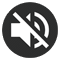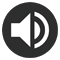# 9 Times table Chart - Printable PDF

11 Reviews

## Print the 9 Times table Chart

A pretty 9 Times table chart in A4 format (PDF) that will help you learn your 9 times table. Thanks to its colored numbers, it will make it easier for you to memorize the multiplication results.

Print for free this 9 times table and don't forget to take a look at the time-saving trick for the 9 times table. Then, practice online this times table. It will help you memorize the table even faster.

This chart is also available in other colors or in black and white. Feel free to look at all the templates we propose below and choose your favorite one!
In a Multiplication Chart, the results are displayed in a table (with columns and rows), whereas in a Times table Chart they are displayed as a list of increasing multiplications.

Here the list starts at 9x1 and ends at 9x12. One way to memorize your table is to recite it aloud in this way:

9 times 1... 9,  9 times 2... 18,  9 times 3... 27,  9 times 4... 36,  9 times 5... 45,  9 times 6... 54,  9 times 7... 63,  9 times 8... 72,  9 times 9... 81,  9 times 10... 90,  9 times 11... 99,  9 times 12... 108

This way of learning using auditory memory is effective for many children.

Print the 9 Times table Chart (PDF)

If you plan to print the other times tables, we advise you to choose a different color for each multiplication table from 1 to 12. To save time, you will find, in our All Times tables section, PDFs including all the tables colored like a rainbow.

## Our other 9 Times table PDF

Thanks to the many colors available, you will undoubtedly find your favorite chart! Our black and white 9 Times table charts will be perfect if you prefer sobriety, if you want to save ink or if you prefer to color your times table yourself using your pencils or markers. Feel free to click on the images to preview the PDF or use the "PRINT" button below the image to download the chart of your choice.

9 Times table Chart
Black & white
Print
9 Times table Chart
Handwritten numbers
Print
9 Times table Chart
Ink saving
Print
9 Times table Chart
Grey
Print
9 Times table Chart
Dark green
Print
9 Times table Chart
Green
Print
9 Times table Chart
Light green
Print
9 Times table Chart
Turquoise
Print
9 Times table Chart
Blue
Print
9 Times table Chart
Dark blue
Print
9 Times table Chart
Purple
Print
9 Times table Chart
Pink
Print
9 Times table Chart
Light pink
Print

## Trick for the 9 times table

This times table may seem difficult, but you'll see that, with the help of your fingers, knowing the table of 9 is a piece of cake! To quickly find all the results in this table, you'll need just your 2 hands and your 10 fingers.

• 1- Place your hands side by side, palms facing you. and imagine that fingers are numbered from 1 to 10 from left to right.
• 2- Bend the finger corresponding to the number multiplied by 9.
• 3- Count the number of fingers to the left of the folded finger and get the first digit of the result.
• 4- Count the number of fingers to the right of the folded finger and get the second digit of the result.
• 5- Put the 2 numbers side by side, you get the result of the multiplication!

Let's take the example of the multiplication 9 x 3:

• 1- Place your hands side by side, palms facing you.
• 2- Plie the doigt correspondant au 3.
• 3- The number of fingers to the left of the folded finger is 2.
• 4- The number of fingers to the right of the folded finger is 7.
• 5- By placing the 2 numbers side by side, you get 27.

### The results of the 9 times table

• 9 x 1 = 9  (see the 1 time table)
• 9 x 2 = 18  (use the finger trick below)
• 9 x 3 = 27  (use the finger trick below)
• 9 x 4 = 36  (use the finger trick below)
• 9 x 5 = 45  (use the finger trick below)
• 9 x 6 = 54  (use the finger trick below)
• 9 x 7 = 63  (use the finger trick below)
• 9 x 8 = 72  (use the finger trick below)
• 9 x 9 = 81  (use the finger trick below)
• 9 x 10 = 90  (see the 10 times table)
• 9 x 11 = 99  (see the 11 times table)
• 9 x 12 = 108  (see the 12 times table)

### 9 x 2

Place your hands side by side, palms facing you. As 9 is multiplied by 2, bend the finger number 2. Next, count the number of fingers to the left of the folded finger (there is 1) and then the number of fingers to the right of the folded finger (there are 8). The result of multiplying 9 x 2 is 18.

### 9 x 3

Place your hands side by side, palms facing you. As 9 is multiplied by 3, bend the finger number 3. Next, count the number of fingers to the left of the folded finger (there are 2) and then the number of fingers to the right of the folded finger (there are 7). The result of multiplying 9 x 3 is 27.

### 9 x 4

Place your hands side by side, palms facing you. As 9 is multiplied by 4, bend the finger number 4. Next, count the number of fingers to the left of the folded finger (there are 3) and then the number of fingers to the right of the folded finger (there are 6). The result of multiplying 9 x 4 is 36.

### 9 x 5

Place your hands side by side, palms facing you. As 9 is multiplied by 5, bend the finger number 5. Next, count the number of fingers to the left of the folded finger (there are 4) and then the number of fingers to the right of the folded finger (there are 5). The result of multiplying 9 x 5 is 45.

### 9 x 6

Place your hands side by side, palms facing you. As 9 is multiplied by 6, bend the finger number 6. Next, count the number of fingers to the left of the folded finger (there are 5) and then the number of fingers to the right of the folded finger (there are 4). The result of multiplying 9 x 6 is 54.

### 9 x 7

Place your hands side by side, palms facing you. As 9 is multiplied by 7, bend the finger number 7. Next, count the number of fingers to the left of the folded finger (there are 6) and then the number of fingers to the right of the folded finger (there are 3). The result of multiplying 9 x 7 is 63.

### 9 x 8

Place your hands side by side, palms facing you. As 9 is multiplied by 8, bend the finger number 8. Next, count the number of fingers to the left of the folded finger (there are 7) and then the number of fingers to the right of the folded finger (there are 2). The result of multiplying 9 x 8 is 72.

### 9 x 9

Place your hands side by side, palms facing you. As 9 is multiplied by 9, bend the finger number 9. Next, count the number of fingers to the left of the folded finger (there are 8) and then the number of fingers to the right of the folded finger (there is 1). The result of multiplying 9 x 9 is 81.

## How to learn your 9 times table ?

Once you've learnt your times table, don't forget to practice! This will help you memorise the 9 times table over the long term. To do this, we suggest two types of exercise: an interactive online exercise or printable exercises to do at home. Choose the method you prefer!

### 1- Practice with this online exercise

In this exercise, you can choose between several settings, so it's up to you to select the ones that suit you best. Here are the available options:

• Number color: By choosing the colorful numbers option, the color code of the table of 9 (dark pink) is applied. This is the same color code as the printable 9 times table chart at the top of the page. The use of color helps memorization, so we highly recommend the colorful mode!
• Number format: you can choose a specific font for the numbers. There are standard fonts, but also school fonts. Select the one that's closest to the one used at school.
• Game mode: random or increasing. When you don't know your table very well, we advise you to start with the "increasing" mode. As soon as you feel more comfortable, try the "random" mode, which is a little more difficult but will help you memorize all the multiplications in the 9 times table.

You'll notice that at the very bottom of the exercise, we display your most common errors. This will help you to know which multiplications are still giving you trouble, and so, which ones you need to keep practicing.
If you like this exercise, you can also practice with the other tables in the All times tables section.

USEFUL !
You can see your more common mistakes below the settings.NUMBERS :
NUMBER FORMAT :
MULTIPLICATIONS :
GAME MODE :

### Your most common mistakes :

You will find here the list of your most common mistakes

### 2- Print the worksheet

Print the PDF worksheet for the 9 times table thanks to the button below and then fill in the answer fields. Whenever you’re not sure of the answer to a multiplication, circle it in red. This way, you know which ones you need to keep practicing. Once you have filled in all the fields, print a Multiplication Chart or use our Online multiplication chart to check your answers and circle all your mistakes in red. If you made any mistakes or had any hesitations, continue practicing with the 9 times table.

Print the Worksheet
9 Times table (PDF)

### A chart with all the times tables (2 PDF to cut and assemble)

A pretty chart with all the times tables to print in A4 format (PDF). We have assigned a different color code for each times table, with a nice color gradient. The colors will help you memorize all your multiplication tables from the 1 to the 12 times table (other colors are available for this resource).

See the Chart